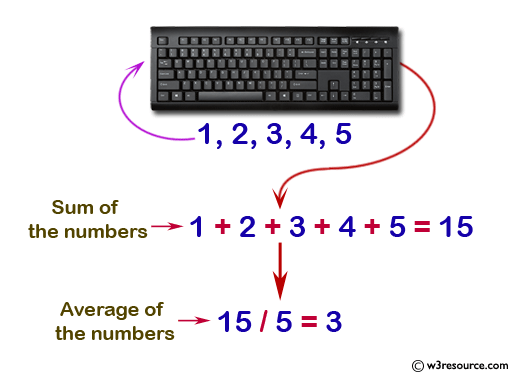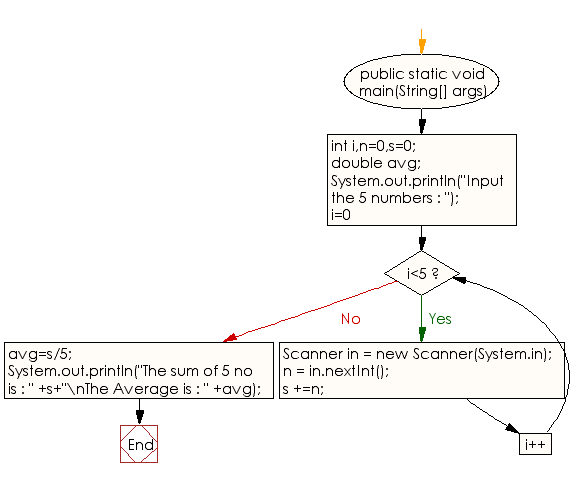﻿ Java exercises: Input 5 numbers from keyboard and find their sum and average - w3resource# Java Conditional Statement Exercises: Input 5 numbers from keyboard and find their sum and average

## Java Conditional Statement: Exercise-12 with Solution

Write a program in Java to input 5 numbers from keyboard and find their sum and average.

Test Data
Input the 5 numbers: 1 2 3 4 5

Pictorial Presentation:Sample Solution:

Java Code:

``````import java.util.Scanner;
public class Exercise12 {

public static void main(String[] args)

{
int i,n=0,s=0;
double avg;
{

System.out.println("Input the 5 numbers : ");

}
for (i=0;i<5;i++)
{
Scanner in = new Scanner(System.in);
n = in.nextInt();

s +=n;
}
avg=s/5;
System.out.println("The sum of 5 no is : " +s+"\nThe Average is : " +avg);

}
}
```
```

Sample Output:

```Input the 5 numbers :
1
2
3
4
5
The sum of 5 no is : 15
The Average is : 3.0
```

Flowchart:Java Code Editor:

What is the difficulty level of this exercise?

﻿

## Java: Tips of the Day

How to split a String by space?

You can use the whitespace regex:

```str = "Hello I'm your String";
String[] splited = str.split("\\s+");
```

This will cause any number of consecutive spaces to split your string into tokens.

Ref: https://bit.ly/3xk42If